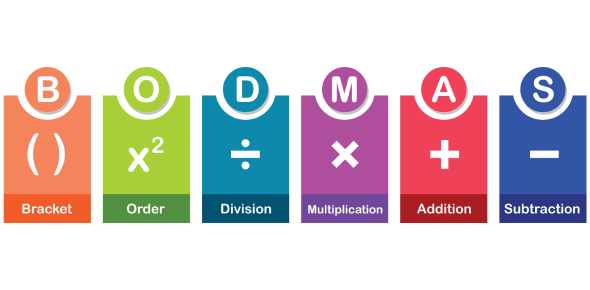# Divisibility Rules Practice Quiz

10 Questions | Attempts: 3982
ShareSettingsYou must have read about the divisibility rules in your maths textbooks. Do you still remember those rules? If yes, play it out this practice quiz and test your knowledge for the same. A divisibility rule is a short and useful method to identify whether any given number is divisible by a fixed divisor without performing the actual division. The below quiz contains ten questions. Let's see how easily you solve it. Shall we begin it now? Good luck!

• 1.
If the sum of the digits of a number is divisible by 3, then the number will be divisible by which of the following?
• A.

3

• B.

9

• C.

2

• D.

7

• 2.
Which of the following number is divisible by 2?
• A.

123

• B.

236

• C.

237

• D.

345

• 3.
1000 can be divisible by which number here?
• A.

5

• B.

3

• C.

2

• D.

2 and 5 both

• 4.
Which of the following number is divisible by both 2 and 3?
• A.

126

• B.

100

• C.

123

• D.

133

• 5.
Which of the following number is divisible by 9?
• A.

78500

• B.

78531

• C.

78532

• D.

78534

• 6.
Which of the following number is divisible by both 3 and 9?
• A.

78531

• B.

78534

• C.

3333

• D.

1234

• 7.
15000 can be divisible by which number here?
• A.

2

• B.

3

• C.

5

• D.

All of the above

• 8.
What numbers are divisible by 2, 3, and 10 at the same time?
• A.

12

• B.

30

• C.

80

• D.

120

• E.

50

• 9.
The number 203 is divisible by 3 and 7
• A.

True

• B.

False

• 10.
36 is divisible by
• A.

10

• B.

5

• C.

2

• D.

7

## Related TopicsBack to top
×

Wait!
Here's an interesting quiz for you.